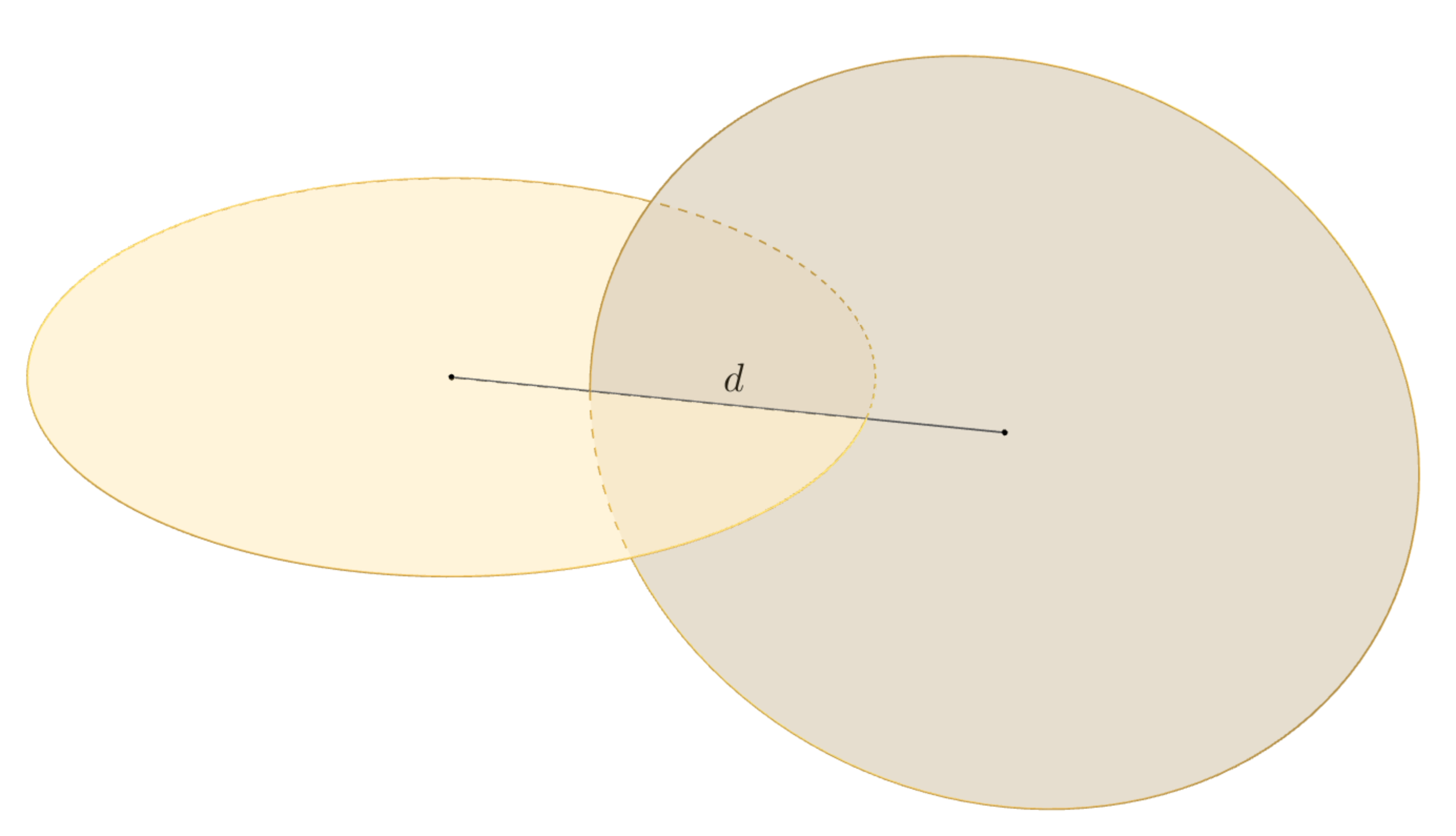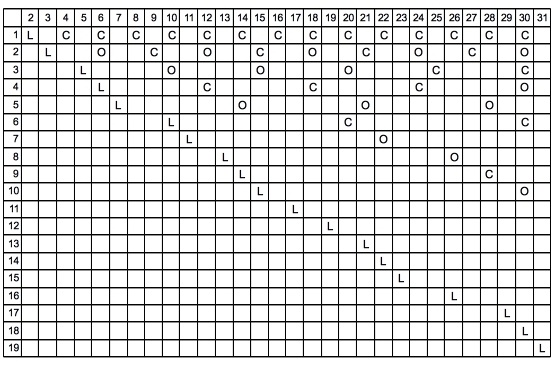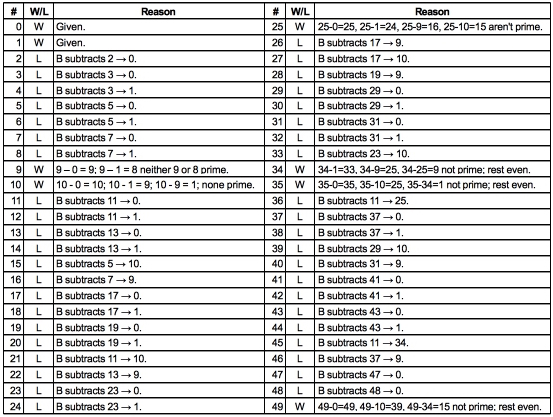###Everything but trains, planes or automobiles — the Varsity Math team is on the go, with suitably unusual means of transportation.Cross Training | Freshman
Five members of the Varsity Math team are running late for a big competition and hurrying toward the arena. Unfortunately, they forgot that they would have to cross the stream that cuts through campus. Fortunately, when they reach the stream, there’s a small boat tied up on their side that they can “borrow” to get to the other side. The boat will hold either one, two, or three of the students, but not more. Fortunately, two of the team members are also on the crew team, and when the boat contains only one or both of them, they can make it across the stream in one minute. Unfortunately, the other three teammates are very inexperienced with boats, and when any of them is aboard, there is so much awkwardness and flailing that the crossing will take four minutes, regardless of whether one of the crew teammates is on board. Unerringly, the team members adopt the fastest strategy for rowing back and forth across the stream to get everyone to the other side for the competition.
How many minutes does it take all five team members to get across?

Sesquicycle | Junior Varsity
Another Varsity Math teammate, Pat, has invented a new transportation device with dreams of revolutionizing how students get around campus. Dubbed the “sesquicycle,” it consists of two circular discs, each one half a meter in diameter, intersecting at right angles as shown in the diagram above. The idea is that a student will stand on the device and step on each disc, alternating left and right in turn, to make the device go forward. (Don’t try this at home!) Now Pat is perplexed, because the sesquicycle prototype doesn’t seem to roll very smoothly. Another teammate provides a helpful remark: “The thing will roll the best when its center of gravity remains at a constant height as it rolls forward.” You realize that you can make that happen by adjusting how long the grooves are that Pat cuts to slide the discs together, which determines the distance (labeled d in the accompanying diagram) separating the centers of the two discs.
How far apart do you advise Pat to place the centers of the discs to make the sesquicycle roll the most smoothly?

### Solutions to Week 5

Now that we are done with the first relay, here are the solutions to all of the problems involved in the relay from the past five weeks:

Urn Respect. Since drawing a single ball at the start has an even chance or producing red or black, the urn must start with the same number of red and black balls. Call that number n. (Our final answer will be 2n, the total of the red and black balls.) If the first two you draw match, then the urn contains n and n-2 of the two colors of balls, respectively. (Note it doesn’t matter which color is which, since the colors of the balls could be any two colors, or could even be swapped. All that matters is the relative number of each kind of ball.)

We’re told that now there’s a 50/50 chance that another pair drawn at random will match each other. We have to figure out the value for n that makes that true. It’s actually easier to calculate the probability that the two balls don’t match, which also has to be 50/50 (because that occurs in exactly the 50% of cases left when you eliminate the cases in which the balls match). To calculate this probability, we need to find the number of pairs that don’t match, and divide by the total number of different pairs of balls from the urn. Since there are n balls of one color and n – 2 of the other, to make a mismatched pair we have to take one of each, and there are n(n – 2) ways to do that.

How many pairs are there in all? Well, there are a total of n + n – 2 = 2n – 2 balls in the urn at this point, and choosing a pair consists of choosing any one of them (2n – 2 possibilities) and then choosing another (2n – 3 possibilities remaining). To get the number of different pairs, however, you have to divide by two because a given pair could have been chosen in either one of two orders. So there are (2n – 2)(2n – 3)/2 = (n – 1)(2n – 3) pairs in all. Thus, the probability of picking a mismatched pair is n(n – 2)/(n – 1)(2n – 3), and we want that to equal 50%. Solving n(n – 2)/(n – 1)(2n – 3) = 1 / 2, we find (by cross multiplying) that 2n(n – 2) = (n – 1)(2n – 3). Multiplying both sides out, that’s 2n2 – 4n = 2n2 – 5n + 3, so that n = 3. Doubling n (as noted earlier), your friend’s urn started with 2n = 6 balls in it.

Carambola Orchard. Let’s call the number of trees in the first category (that are in three rows each) k, and the number of trees in the second category (in five rows each) l. What should we try to do with those numbers k and l? Well, we could try to count the rows. For example, if k of the trees are on 3 rows each, then it seems like that accounts for 3k rows, and if l trees are on 5 rows each, that seems to account for 5l rows, so there should be 3k + 5l rows in all, right? Well, that would be right except that we’ve counted every row four times: since there are four trees in each row, each row gets “noticed” by four different trees when we add things up from the trees’ point of view. So we have to divide 3k + 5l by 4 to get the number of rows, or in other words, we’ve deduced that (3k + 5l)/4 = 15. So that’s one equation between our two unknowns; where can we get another? Just from the total number of trees: if there are two categories of trees with k and l trees respectively, then k + l has to be the total number of trees, which is 16. Now we have two equations – multiplying the first equation by 4, we have 3k + 5l = 60 and k + l = 16. Subtracting three times the second equation from the first, we get 2l = 12, so l = 6. Since 6 matches the answer to Urn Respect, that’s the category we are looking for—and looking back at the definition of l above, that’s the letter we used for the number of trees in five rows, so the answer to this problem is 5.

Bonus answer: Despite the coach’s tip, some of you must be wondering how to arrange those trees. Well, for a hint, you might want to look up what grows on a carambola tree…

Freshman Tip from Coach Newton:
Hey, team! Don’t expend your energy answering a question the problem didn’t ask. In this Orchard problem, nobody said you had to figure out how to arrange the trees to have all these rows like this. That’s pretty hard to do, so maybe see if you can solve the problem that is asked without getting in a fuss about where exactly all those trees go. Maybe it’ll all work out. If it doesn’t, you can always try to draw the diagram as a fallback… Good luck out there!

Rush Job. The maximum paint that can be left over is just enough to cover the maximum surface area that can be hidden by gluing the blocks together. Since each glue area is a planar surface of one of the blocks, each glue joint formed can cover at most twice the area of the largest planar portion of the surface of a block (since the glue joins two wooden surfaces). The largest planar area of one of these blocks is the biggest face, 8 cm by 18 cm, for 144 sq. cm. Usually, two glue joints are formed to join three blocks together; each one hides at most 288 sq. cm. (There are some interesting configurations in which there are three glue joints, so that each block has glue on two different faces, but you can easily check that these configurations sacrifice a lot of glue area. That’s because in these configurations, no block can have glue on both of its large sides.) Hence, the largest amount of surface area that can be joined is 576 sq. cm. Dividing by the paint coverage, 96 sq. cm. per milliliter of paint, Larry will have at most 6 milliliters of paint left.

JV tip from Coach Newton:
Hey, notice that only the 8 cm by 18 cm side comes into the solution? Either of the possible numbers that could have come from Carambola Orchard into this round of the relay is small enough so that it won’t affect the outcome of Rush Job. That happens sometimes in relays, and it means you can solve a stage even if you didn’t solve the prior stage. Keep your eyes sharp for this!

Unsustainable Agriculture. Let’s just say each cow eats one unit of grass per day. The grass grows at some rate r units per day, and there is some amount, say g units of grass, already in the pasture to begin with. The pasture is depleted when the amount of grass eaten equals the starting amount of grass plus the grass that has grown so far. So for 6 cows in 25 days, that’s 150 units of grass eaten, which equals g units plus 25r units of grass; and for 7 cows in 13 days, that’s 91 units of grass eaten, which equals g units plus 13r units of grass. Subtracting the second equation from the first, 59 = 12r, or r = 59/12 units of grass per day. Plugging back into 91 = g + 13r, we get g = 91 – 13(59/12), or g = 325/12. Therefore, in one day, there are 325/12 + 1(59/12) = 384/12 = 32 units of grass available to eat, so it will take 32 cows to eat that all up.

A more elegant solution from Wall Street Journal reader David Moulton: The net effect of A cows grazing and the pasture growth is to deplete 1/25 of the original supply of grass per day. The net effect of (A + 1) cows is to deplete 1/13 of that supply per day. Therefore, the additional effect of one cow is to deplete 1/13 – 1/25 = 12/325 of the original supply per day. To further increase the rate of depletion to 1 entire amount of the original supply per day (i.e., deplete the field in a single day), you need (1 – 1/13) / (12/325) = (325/325 – 25/325) / (12/325) = 300 / 12 = 25 additional cows. Since A from Rush Job is 6, you need A + 1 + 25 = 32 cows to polish off the field in one day.

Bonus question: How long will the pasture support five cows? In the notation of the first solution above, we are looking for a number of days d such that 5d = 325/12 + (59/12)d. Multiplying by 12, that’s 60d = 325 + 59d, which simplifies to d = 325 days — amazing what a difference one fewer cow makes. What happens with fewer than five cows, and why?

Laborious Lockup. Consider this table of what happens to the first 30 lockers in the first 19 runs (the first column is the run number, and L stands for Locked, C for Closed, and O for Opened):After this point, nothing further happens to the first 31 lockers; all the action is off to the right. So these are the final states of all of these lockers. So we see that the following locker numbers end up locked: 2, 3, 5, 6, 7, 10, 11, 13, 14, 15, 17, 19, 21, 22, 23, 26, 29, 30, 31, and the other lockers end up closed but not locked: 4, 8, 9, 12, 16, 18, 20, 24, 25, 27, 28. The presence of 4, 9, and 25 in the second list suggests that it has something to do with squares, and a little more poking around reveals that the second list (closed but not locked) contains all the numbers that have a factor which is a square, and the other list (the locked ones) contains the numbers that have no factor which is a square — the so-called square-free numbers. The pros among you can prove the property always holds — it turns out that it does — and that now lets us figure out what happens at the end of the hallway. The last locker in the hallway is 848, and it turns out that all of 848, 847, 846, 845, and 844 are divisible by squares, whereas 843 = 3 × 281 is not. So 843 is the last locked locker.

Bonus question: Why 847 lockers? Bonus answer: Because that way there are just enough lockers so that you get to the very first occurrence of five numbers in a row, all of which are divisible by squares.

Prime Event. First note that if Anna were allowed just to play either a 0 or a 1, that would be an instant win. On the other hand, if she started with any number from two through eight, Basil would just subtract 2, 3, 5, or 7 to produce 0 or 1 and win. So those are losing numbers for Anna to start with. If she started with a nine, then Basil’s only plays would be to subtract 2, 3, 5, or 7, leaving results in that two through eight zone, setting Anna up to produce 0 or 1 on her next turn. So nine is a winning number for Anna to start with. You can continue this style of reasoning to determine for each successive number whether it is a losing choice for Anna or a winning choice. If any winning choice is a prime number less than the number you’re considering, then that number is a losing choice (because then Basil will go to that winning choice and Anna will be stuck). On the other hand, if every number you can reach by subtracting a prime is a losing choice, then that will be a winning choice for Anna, because Basil will have to go to a losing number on his turn. Looked at the other way, if all of the lower winning choices are a non-prime number away, then the new number is a winning choice. The fact that all primes except two are odd makes this calculation reasonably quick to do, even by hand. Here’s a table that runs up to 49, well past what’s needed to find the first winning choice bigger than 32:Consulting the table, we see that 34 is the lowest winning choice larger than 32.

And on the bonus question, you can see above that 9, 25, and 49 are winning choices for Anna. These are the squares of the first three odd primes. But don’t be fooled! If you keep extending the table (most likely with the help of a computer program), you will find that 529, which is the square of 23, is not a winning choice. That’s the first odd prime square that’s not a winning choice.

### Previous Weeks

Week 5: Laborious Lockup & Prime Event, solutions to Week 4
Week 4: Unsustainable Agriculture & Save the Camel, solutions to Week 3
Week 3: Deranged Assistant & Rush Job, solutions to Week 2
Week 2: Carambola Orchard & Farm Roundup, solutions to Week 1
Week 1: Instant Interrogation & Urn Respect

Find more puzzles at varsity.momath.org.